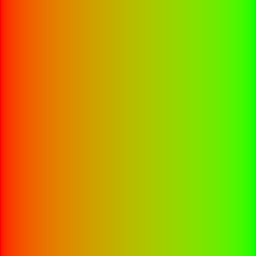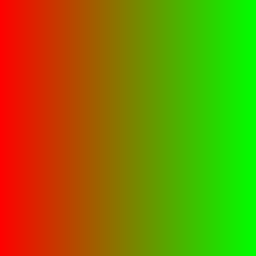# Color Gradients¶

This page covers functions with the `heman_color_` prefix.

One-pixel tall images can be interpreted as gradients, also known as lookup tables.

## Creating Gradients¶

Clients can import a gradient using `heman_import_u8`, or they can create one from scratch:

heman_image* `heman_color_create_gradient`(int width, int num_colors, const int* cp_locations, const heman_color* cp_colors)
Create a 1-pixel tall, 3-band image representing a color gradient that lerps the given control points.
Parameters: width (int) – Desired number of entries in the lookup table. num_colors (int) – Number of control points. Must be at least 2. cp_locations (int*) – The X coordinate of each control point. The first value must be 0 and the last value must be width - 1. cp_colors (heman_color*) – The RGB values of each control points.

## Gamma Correctness¶

The following two images both depict the interpolation of (1,0,0) to (0,1,0). Can you tell which one is more correct?The image on the left is more correct; it interpolates the colors by first unapplying gamma to the control point colors, then performing linear interpolation, then re-applying gamma. Heman does this automatically when you call `heman_color_create_gradient`, but the behavior can be controlled with the following function.

void `heman_color_set_gamma`(float f)

This sets some global state that affects lighting and color interpolation. The default value is 2.2.

## Applying Gradients¶

heman_image* `heman_color_apply_gradient`(heman_image* heightmap, float minheight, float maxheight, heman_image* gradient)

Create a 3-band image with the same dimensions as the given heightmap by making lookups from a 1-pixel tall color gradient. The heightmap values are normalized using the given minheight, maxheight range.